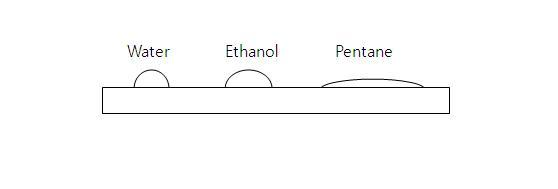# A chemistry problem by Gandhali Rangan

Chemistry Level 1The above figure shows the cross sectional shape of water, ethanol and pentane when you drop an equal volume of each liquid on a glass plate. Which of the following orders regarding $\Delta H_{\text{vapor}}$ is correct?

×